TC, BN, JBM, AZ

# Motivation¶

Matplotlib is a general 2D / 3D plotting library used in many Python scientific libraries. It is used in Pandas and Scikit-learn. Its syntax is quite consistent and allows one to create numerous high-quality figures

Resources: http://matplotlib.org/

## Installation¶

conda install matplotlib

# Convention¶

import matplotlib
import matplotlib.pyplot as plt
# OR
import pylab


## Using matplotlib¶

#### 1. In the notebook, type this command to import all functionalities and include images in the notebook automatically:¶

In :
%pylab inline

Populating the interactive namespace from numpy and matplotlib


#### 2. In a script or shell:¶

import pylab


#### 3. In ipython, starts ipython as follows::¶

ipython --pylab

### Notes for fine tuning¶

In :
import matplotlib
matplotlib.rcParams['figure.figsize'] = (10,7.5)


# A toy data set using Bioservices and Pandas¶

### A dataframe with drug/compound information¶

In :
# pip install bioservices should work
from bioservices import ChEMBL

In :
chembl = ChEMBL()

In :
Ncomp = 10
res = chembl.get_compounds_by_chemblId(
['CHEMBL%s' % i for i in range(Ncomp)])

In :
# some results are not found and tagged as 404 integer; let us remove them
res = [x for x in res if x != 404]

In :
res

Out:
{'compound': {'acdLogd': 7.67,
'acdLogp': 7.67,
'alogp': 3.63,
'chemblId': 'CHEMBL1',
'knownDrug': 'No',
'molecularFormula': 'C32H32O8',
'molecularWeight': 544.59,
'numRo5Violations': 1,
'passesRuleOfThree': 'No',
'rotatableBonds': 2,
'smiles': 'COc1ccc2[C@@H]3[C@H](COc2c1)C(C)(C)OC4=C3C(=O)C(=O)C5=C4OC(C)(C)[C@@H]6COc7cc(OC)ccc7[C@H]56',
'stdInChiKey': 'GHBOEFUAGSHXPO-XZOTUCIWSA-N'}}
In :
# select compound to make things easier
res = [x['compound'] for x in res]

In :
import pandas as pd
df = pd.DataFrame(res)


Obtaining compounds from ChEMBL takes time. In the example above, we look for only 10 compounds. However, you can find files in JSON and CSV files in ./data/chembl.json and ./data/chembl.csv with 5,000 compounds.

# In pure Python, JSON can be loaded as follows:
import json

# With Pandas, you may use
import pandas as pd

In :
df = pd.read_csv("data/chembl.csv")
df = df[["alogp", "molecularWeight"]]
df.dropna(0, inplace=True)
X, Y = df.alogp, df.molecularWeight


See ChEMBL notebook and Pandas notebook for more details about using Pandas

# Quick way to get the sample data set¶

In :
import numpy as np
X = data[:,0]
Y = data[:,1]


# Some concepts and terminology# Concepts and terminology¶

### Figure¶

The figure is like a canvas where all your Axes (plots) are drawn. A figure can contain several Axes. For now, we will use only one.

### Axes¶

This is what you think of as ‘a plot’.

• The Axes contains two (or three in the case of 3D) Axis objects
• Each Axes has a title
• Each Axes can contain a legend

### Axis¶

These are the number-line-like objects.

### Labels¶

This is the "legend" of Axis. There are 2 labels for 2D plots the x_label and y_label.

### Ticks¶

The ticks are the marks on the axis and ticklabels (strings labeling the ticks). There are two kind of ticks: major and minor ticks. By default they are automaticaly generated by the axis but they can be configured.

## Coding Styles¶

Matplotlib has two coding styles:

• matlab style (functional)
plot(...)
xlabel(...)
title(...)

• vs object-oriented approach
fig = figure()
ax.plot(...)
ax.xlabel(...)
ax.title(...)

The two styles are perfectly valid and have their pros and cons.

# Matplotlib by examples

## The plot function (2 variables)¶

In :
plot(X, Y)

Out:
[<matplotlib.lines.Line2D at 0x7f63ada6e588>]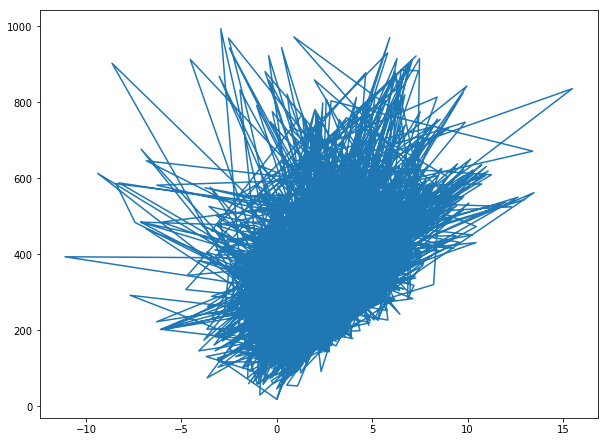In :
# Let us make it nicer by providing a marker:
plot(X, Y, marker='o')

Out:
[<matplotlib.lines.Line2D at 0x7f63aea584a8>]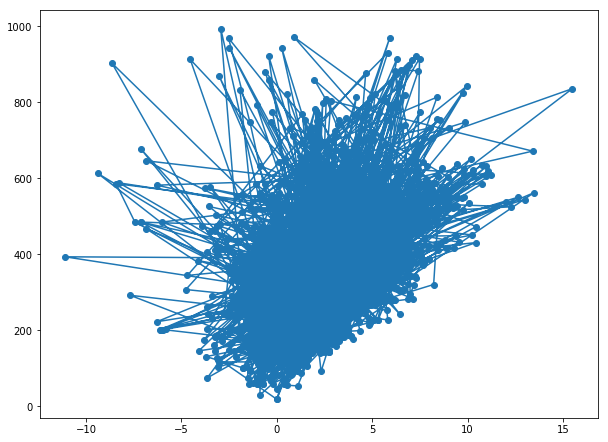In :
# let us also remove the lines
plot(X, Y, marker='o', linestyle='')

Out:
[<matplotlib.lines.Line2D at 0x7f63add86860>]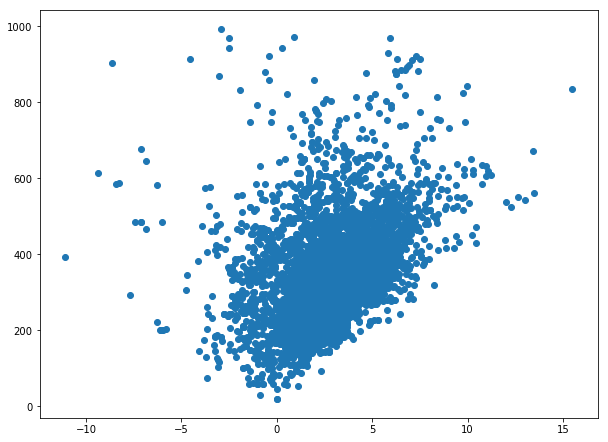In :
# an alias to the marker and linestyle is to use a third positional
# argument. Here b means blue color, o means circle marker and no
# third letter is provided for the style
plot(X, Y, 'bo')

Out:
[<matplotlib.lines.Line2D at 0x7f63c0be4208>]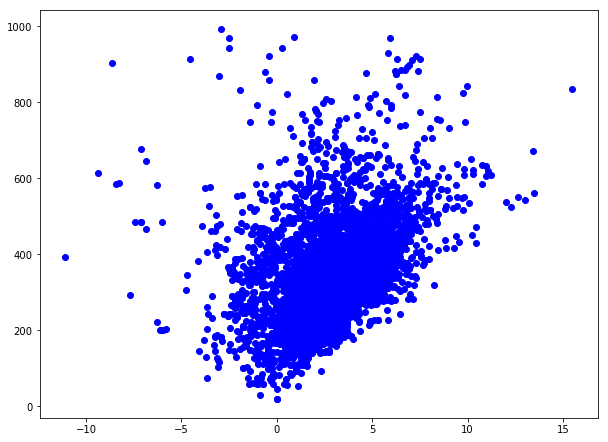look into the doc to figure out

• the color (e.g., k for black, r for red,...)
• the marker (e.g., o for circles, x for crosses, s for square)
• the line style (e.g., -- for lines, - for dashed lines)
In :
plot(X, Y, color='red', marker='s', linewidth=0)

Out:
[<matplotlib.lines.Line2D at 0x7f63b20ce518>]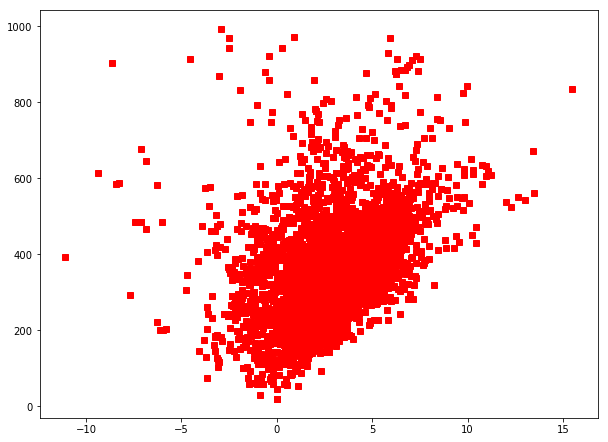In :
plot(X, Y, color="brown", marker="v", lw=0)

Out:
[<matplotlib.lines.Line2D at 0x7f63aea4bd30>]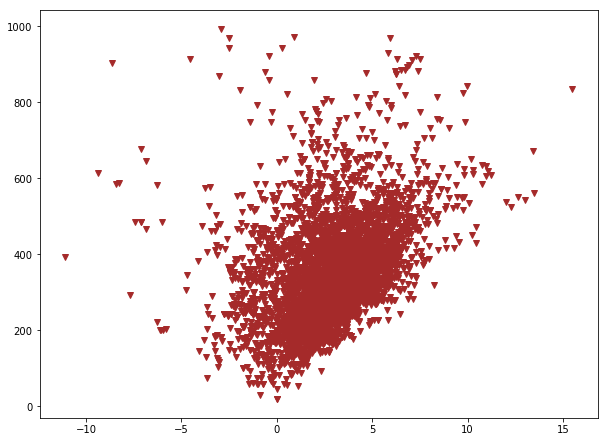## Plot function (1 variable) and the hold function¶

In :
# You can provide just 1 variable as an input to the plot function:
plot(X, "b")

Out:
[<matplotlib.lines.Line2D at 0x7f63aeaed2b0>]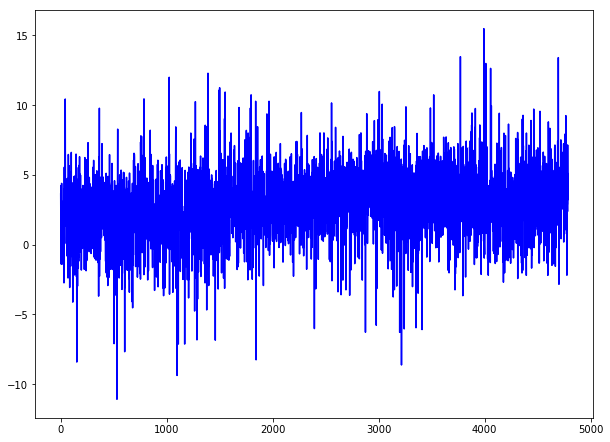In :
plot(X, "b")
# hold is True by default
plot(Y/20, "r")

Out:
[<matplotlib.lines.Line2D at 0x7f63ae9ecfd0>]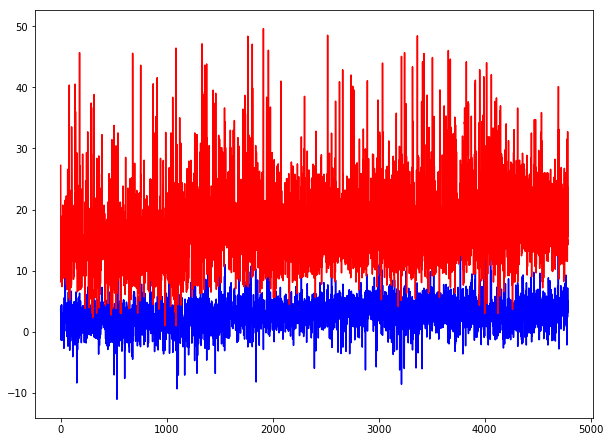In :
plot(X, "b")
#hold(False)  # deprecated
clf()
plot(Y/20, "r")

Out:
[<matplotlib.lines.Line2D at 0x7f63aeaa1400>]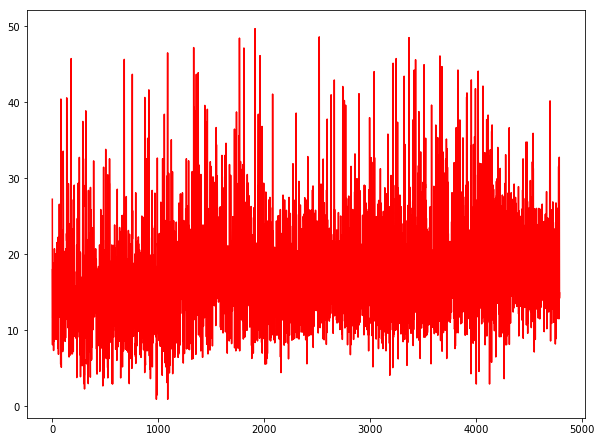## xlabel and ylabel¶

In :
# note that color may be provided as hexadecimal values
plot(X, Y, 'o', markersize=8, color='#ffaa11')
xlabel('alogp')
ylabel('molecularWeight')

Out:
<matplotlib.text.Text at 0x7f63ae9c1d30>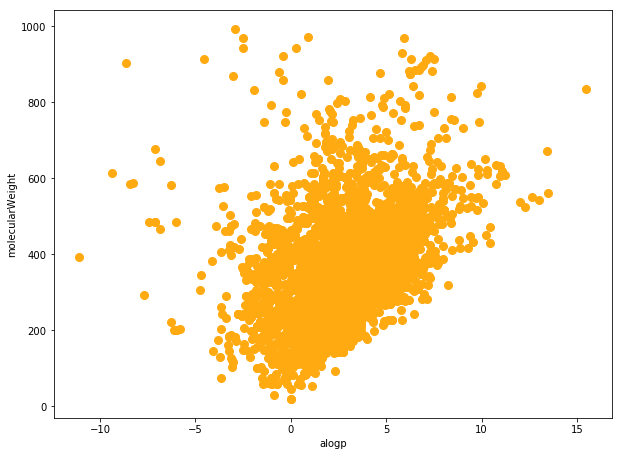In :
# just an alias for later.
x = linspace(0,10,1000)
y1 = cos(4 * 3.14159 * x / 10)
y2 = 0.4*cos(8 * 3.14159 * x / 10)
plot(x, y1+y2)
xlabel('$Y(t)$', fontsize=16)
# Note r"" is for raw string to have more robust LaTeX code
_ = ylabel(r'$\sum_i^N Y(t)= \sum_i^N \cos{(\frac{\pi t )}{10} ) }$',
fontsize=16)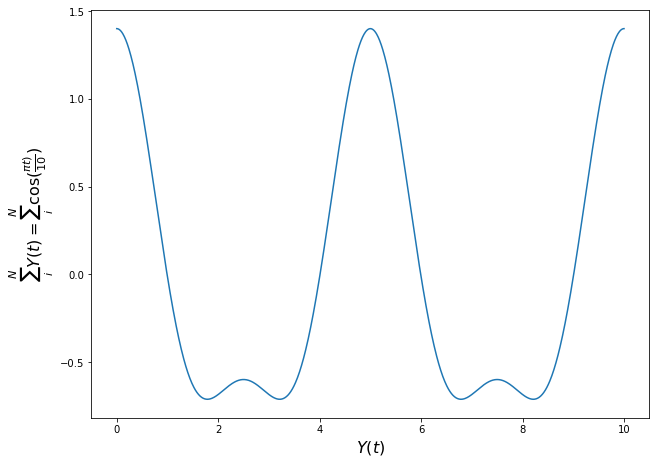## grid function; markersize, fontsize, alpha parameters¶

In :
# mec -> markeredgecolor
plot(X, Y, 'o', markersize=8, mec="k")
xlabel('alogp')
ylabel('molecularWeight')
grid(True)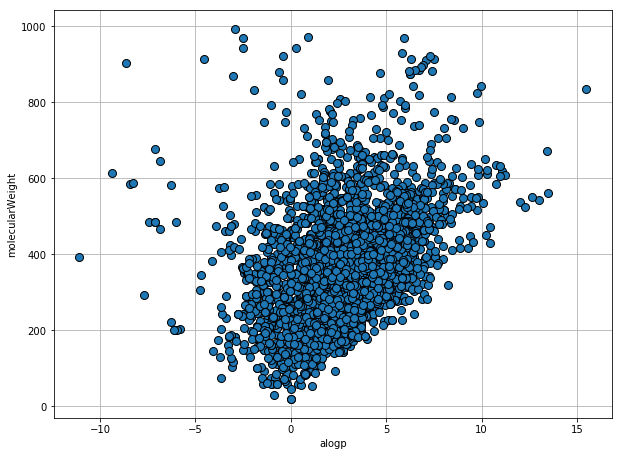In :
# a bit more tuning on the grid and fontsize
plot(X, Y, 'o', markersize=8, alpha=0.5, mec="k")
xlabel('alogp', fontsize=20)
ylabel('molecularWeight',fontsize=25, color='red')
grid(color='r', linewidth=2, linestyle='--', alpha=0.5)